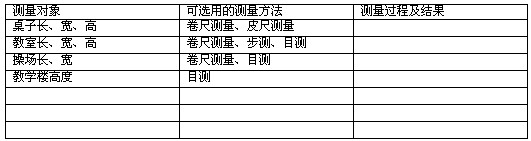# 西师版小学一年级数学教案2021

2021西师版小学一年级数学教案1

1.在游戏活动中，帮助学生掌握有关“6”和“7”的加减法.

2.在游戏活动中，鼓励学生积极参与、积极交流、积极思考，并培养学生有序思维的能力.

3.在游戏活动中，使学生不断积累经验，发展他们的数感.

(一)猜数“2”或“4”

1.教师谈话：我们一起玩一个猜数游戏好不好?(教师出示一个磁珠，让学生看看它的大小)猜一猜老师的两只手里一共抓了几个这样的磁珠?

2.学生猜数，并说出简单的理由.

3.教师提问：

(1)老师的手里到底有几个磁珠哪?想不想知道?

(2)看看老师的`左手有几个?(教师把左手的4个磁珠贴在黑板上)

(3)右手哪?(教师把右手的2个磁珠贴在黑板上)

(4)有谁猜对了?你怎么知道一共有6个磁珠呀?

(左手有4个磁珠，右手有2个磁珠，合起来一共有6个.)

(5)你能用数学算式表示吗?

2+4=6 4+2=6

4.教师谈话：还想不想再玩一次?我们还用这6个磁珠，(教师把这六个磁珠摘下，重新握在手里)老师的两只手里都有磁珠，如果告诉你一只手里有几个，你能猜出另一只手里有几个吗?(教师按照学生的意愿出示一只手中的磁珠的数量2或4)谁能猜出我的另一只手中有几个?

5.教师提问：

(1)你猜对了吗?你怎么那么肯定你猜对了?

(2)能把你的想法用数学算式表示出来吗?

2+4=6 4+2=6 6-2=4 6-4=2

6.小结：你们猜得有理有据，所以都猜对了，快为你们的胜利鼓鼓掌吧!

(二)猜数“3”

1.我们还用这6个小磁珠，换个玩法好不好?(教师用手捂住3个)猜猜老师用手捂住了几个?

2.你能用数学算式表示吗?3+3=6 6-3=3

(三)猜数“1”和“5”

1.还是这6个磁珠，谁愿意当小老师带大家玩一玩，(教师悄悄地引导请上来的学生捂住1个)猜一猜他捂上了几个?

1+5=6 5+1=6 6-1=5 6-5=1

2.你们是不是都很想玩猜数游戏?那同桌的两位小朋友就来一次猜数大赛好不好?

(一)教师谈话：同学们从学具盒里数出7个小珠子，看谁数得快!

(二)教师说明游戏规则

(三)小组活动.

(四)你们俩是怎样玩猜数游戏的，结果怎样?

0+7=7 7+0=7 7-0=7 7-7=0

1+6=7 6+1=7 7-1=6 7-6=1

2+5=7 5+2=7 7-2=5 7-5=2

3+4=7 4+3=7 7-3=4 7-4=3

(五)小结

3+4= 7-4= 7-3= 7-5= 1+6= 2+5=

6-3= 7-1= 7-7= 4+2= 3+3= 1+5=

(一)出示图片：连一连

(二)教师提问：看一看，谁看明白了?(把加起来和是7的数连起来.)

(一)出示图片：老鼠背土豆

(二)学生自编故事

(三)能根据他们编的数学故事列出数学算式吗?

0+7=7 7+0=7 7-0=7 7-7=0

1+6=7 6+1=7 7-1=6 7-6=1

2+5=7 5+2=7 7-2=5 7-5=2

3+4=7 4+3=7 7-3=4 7-4=3

(四)小结：看到你们这么聪明、能干，小老鼠自己却不劳动，偷东西吃，心里非常难过，他表示以后一定改邪归正，自食其力.

2021西师版小学一年级数学教案2

1.鼓励学生算法多样化，培养和激发学生的创新意识.

2.培养学生主动获取新知识和自主探究的能力.

3.掌握十几减9的口算方法，提高学生的计算能力.

1.出示图片：蛋糕1

2.思考：左边有一整块，右边有一小块，明明说他吃半块就能够吃饱，可以怎样切呢?

(1)把整块蛋糕切成两半拿走半块;

(2)把整块蛋糕切成四份，拿一份和另外的一小份组成半块.

3.小结：同学们真聪明，能用不同的方法来解决问题.

4.出示图片：蛋糕2

5.出示图片：蜡烛

6.出示图片：气球

(一)学习“14-9”的口算方法.

1.教师提问：“14-9”等于几，可以怎样算?

2.学生反馈

(1)方法一：因为9+5=14，所以14-9=5

(2)方法二：先用10-9得1，再用1+4=5

(3)方法三：14先减4，再减5.

(4)方法四：14先减10，再加1.

3.小结

(二)学习“17-9”的口算方法.

1.教师谈话

2.小组交流

17-9可以怎么想呢?把你们的想法和小组的同学说一说，看看哪个小组想的又好，说的又清楚!

3.教师巡视指导.

4.学生反馈

5.揭示课题

14-9、17-9这两道题大家已经学会，这实际上已经研究了十几减9的方法了.

6.编题练习

1.讲明游戏规则，帮助欢乐球找到家.

2.小组活动

1、创设情景，激发兴趣。《新课标》指出：数学教学必须注意从学生的生活实际和感兴趣的事情出发，为学生提供观察和实践的机会，体会数学就在身边，感受学习数学的乐趣。本课通过创设“过生日”的问题情景，激发了学生的学习兴趣，再通过老师巧妙地设疑，把生活中经常遇到的问题转化为数学中的加减法，帮助学生学会从数学的角度提出问题，理解问题。

2、体现算法多样化。教师给予学生充分的民主自由，鼓励学生用已有的经验大胆思维，鼓励学生动手操作，寻求解决问题的途径，课堂气愤宽松、活跃。在计算过程中，体现了以学生为主的教学原则，培养了创新思维。

2021西师版小学一年级数学教案3

1.借助熟悉的物体，使学生正确用数表示20以内这些物体的个数.

2.使学生根据11至20个数的组成，掌握20以内不进位加法和不进位减法的计算方法.

3.培养学生动手操作、善于思考的能力.

(一)搭积木(出示图片：说一说1)

1.同学们，你们喜欢玩搭积木的游戏吗?

2.明明和丁丁搭好了两摞积木在列算式时遇到了困难，你们能帮帮他们吗?

3.根据图意谁能列出相应的算式?

4.为什么这样列算式?说一说你的想法.

(1)明明搭了两摞积木，左边一摞是十块，右边一摞是三块，明明又放上了两块一共是十五块，所以就是10+5=15.

(2)丁丁也搭了两摞积木，左边一摞是十块，右边一摞是十八块，拿走了两块是十四块，所以就是16-2=14.

(二)小结

(一)动手操作

1.用你最喜欢的方式表示一个十(画图、用学具盒里的东西或其它物体都可以).

2.请你任意添加一个图或其它物体的个数，组成一个算式，看谁组的多.

3.小组内交流，说一说图意和算式是怎样组成的(出示图片：小棒、三角形).

10+1=11

11-1=10

11-10=1

10+6=16

16-6=10

16-10=6

10+7=17

17-7=10

17-10=7

4.任意举出一列说一说计算的方法

11，十一是由一捆零一根组成的`，拿走了一根还剩下十根所以算式是11-1=10，十一是由一捆零一根组成的，拿走了十根还剩下一根，所以算式是11-10=1.

(二)教师小结

(一)找规律

1.根据我们列出的算式进行整理，你们能找到这些算式计算的规律吗?

2.小组合作交流.

3.指名列出算式，集体反馈.

4.学生汇报，教师板书.

(二)教师小结

(一)出示图片：说一说4

1.请同学们仔细观察.你会得到什么结果?

2.根据相碰的情况列出加法算式.

(二)出示图片：说一说5

1.请同学们仔细观察.你会得到什么结果?

2.根据相碰的情况列出减法算式.

(三)教师小结

2021西师版小学一年级数学教案4

1.通过创设情境，激发学生的学习热情并乐于探究.

2.通过动手操作，让学生在探索相关的退位减法的过程中，进一步感知解题策略的多样化，培养学生创造性思维的意识.

3.通过整理算式，培养学生归纳、概括的能力及良好的思维习惯.

1.出示图片：飞行表演

2.边观察边思考：你看到了什么?想到了什么?

……

(一)活动二：寻找生活中数学问题.

1.根据同学们提出的数学问题，列出相关算式，写在本上.

2.学生汇报，说明算式的含义，并对算式进行分类、整理.

(二)活动三：重点研究11减几的算式.

1.学生汇报，体现解题策略的多样化.

10一7=3 3+1=4

11-1-6=10-6=4

11-10+3=1+3=4

(三)活动四：培养思维有序性

11-2=9 11-9=2

11-3=8 11-8=3

11-4=7 11-7=4

11-5=6 11-6=5

11-6=5 11-5=6

11-7=4 11-4=7

11-8=3 11-3=8

11-9=2 11-2=9

(一)看图列式(出示图片：小鸟和小鸭子)

(二)看图列式(出示图片：松鼠和小兔子)

(三)出示图片：小猫钓鱼

(四)出示图片：小熊射门

1.使学生能熟练计算20以内的加减法.

2.通过游戏，激发学生学习数学的兴趣.

1.以班级为单位活动.

2.教师任意说一个20以内的数，第一个学生任意说一个运算符号(加号或减号)第二个学生再说一个数，第三个学生根据前面所说计算出结果.

3.以第三个学生所说的结果作为加数或者被减数，第四个学生再任意说一个运算符号，第五个学生任意说一个数，第六个学生根据前面所说的计算出结果.

4.后面的学生照此继续进行游戏.

1.学生计算错误或者出的题目超出目前所学范围(例如出现19+9或者6-7这个错误)都要为同学表演节目.

2.要灵活使用这个游戏，变化着练习.

2021西师版小学一年级数学教案5

1.通过问题解决，让学生学会“8+?”的进位加法，初步体会计算方法的多样化，并能在实际中应用理解.进一步理解“凑十法”的含义.

2.组织学生在交流、合作中获得知识和能力.

3.对学生进行环境保护教育

1.出示图片：主题图

2.教师谈话

(一)教学“8+?”

1.根据问题，同学自己思考.

2.小组交流.

3.全班讨论.(出示图片：说一说1和说一说2)

(二)比较

1.计算对比

9 + 6 = 15

8 + 6 = 14

2.口算

9+9 = 9+8 = 9+7 = 9+6 =

9+5 = 9+4 = 9+3 = 9+2 =

8+9 = 8+8 = 8+7 = 8+6 =

8+5 = 8+4 = 8+3 =

3.小组交流心得.

4.全班交流小结.

9加几就减1，8加几就减2.

5.反馈练习.比一比，谁算得快.(说一道，算一道，并说算的`方法)

8+8 7+8 8+4

5+8 8+6 8+3

8+5 2+8 4+8

8+7 9+8 8+9

3+8 6+8 8+2

(一)出示图片：摆一摆，算一算

(二)出示图片：蝴蝶

(三)出示图片：蜻蜓

(四)组织学生到生活中寻找蕴含的数学问题(如：种树问题、游戏问题、银行问题)，

7+( ) 6+( )

#### 西师版小学一年级数学教案2021

教学最重要的任务是：让学生学会怎样学习和怎样思考。要鼓励学生独立思考，有根据的怀疑，敢于和善于提出问题，引导和发展学生的求同和求异思维。可采用讨论法、发现法和自学研究法等。今天小编在这里整理了一些20
推荐度：点击下载文档文档为doc格式

#### 相关推荐一年级下册数学课教案文案

认真编写教案是提高教学水平的重要过程。教师编写教案是一个研究教学计划或教学大纲、教材、教学内容、学员及教法等因素的综合过程。今天小编在这里整理了一些2021一年级下册数学课教案文案，我们一起来看看吧!一年级下册数学捉迷藏教案模板

教学过程中教案是参照系，可以提示教学内容、重点、难点、目标、思路，帮助教师有效完成每一次教学。今天小编在这里整理了一些一年级下册数学捉迷藏教案2021模板，我们一起来看看吧!一年级下册数学捉迷藏教案

热门排行
• 文章标题

• 上篇下篇

• 相关推荐